Function Repository Resource:

# MetricNumberString

Convert raw numbers into metric system amounts for human readability

Contributed by: Michael Sollami
 ResourceFunction["MetricNumberString"][x] returns a human-readable version of the given number x.

## Details and Options

ResourceFunction["MetricNumberString"] uses the standard metric system (SI) prefixes.
ResourceFunction["MetricNumberString"] always returns a formatted string.
ResourceFunction["MetricNumberString"] takes the following options:
 "Decimals" Automatic the number of decimal places after the decimal point NumberPoint "." the decimal point character; e.g. in South Africa you might use a comma "PrefixFunction" Identity custom function which is given the computed prefix and formats a string "PrefixShort" True use short prefixes instead of long; e.g. "m" for "mega" "Spacer" " " string that goes in between the number and unit

## Examples

### Basic Examples (1)

Convert a number to a readable approximation with metric prefixes:

 In:=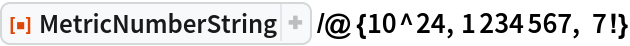Out=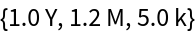### Scope (1)

MetricSize gives unique prefixes up to an octillion (1027):

 In:=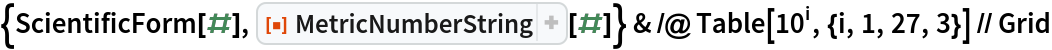Out=### Options (4)

Control the number of digits after the decimal point:

 In:=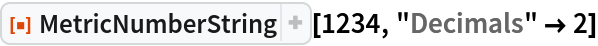Out=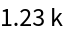Use long prefixes:

 In:=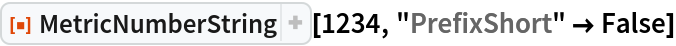Out=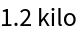Change the spacing between the number and unit:

 In:=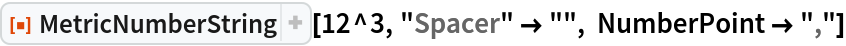Out=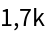Change the label formatting:

 In:=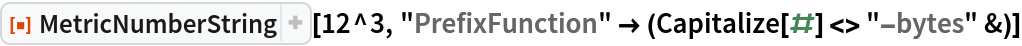Out=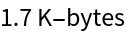### Applications (1)

Display memory in use in human-readable form:

 In:=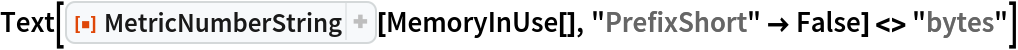Out=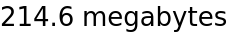### Possible Issues (1)

MetricSize will round in some cases, unless all decimals are asked for:

 In:=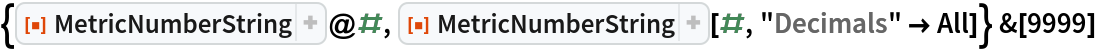Out=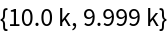Michael Sollami

## Version History

• 2.0.0 – 25 March 2020
• 1.0.0 – 13 January 2020